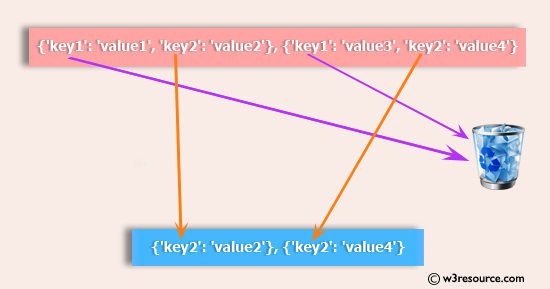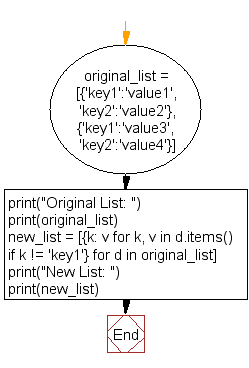﻿ Python: Remove key values pairs from a list of dictionaries - w3resource# Python: Remove key values pairs from a list of dictionaries

## Python List: Exercise - 55 with Solution

Write a Python program to remove key values pairs from a list of dictionaries.Sample Solution:-

Python Code:

``````original_list = [{'key1':'value1', 'key2':'value2'}, {'key1':'value3', 'key2':'value4'}]
print("Original List: ")
print(original_list)
new_list = [{k: v for k, v in d.items() if k != 'key1'} for d in original_list]
print("New List: ")
print(new_list)
```
```

Sample Output:

```Original List:
[{'key1': 'value1', 'key2': 'value2'}, {'key1': 'value3', 'key2': 'value4'}]
New List:
[{'key2': 'value2'}, {'key2': 'value4'}]
```

Flowchart:## Visualize Python code execution:

The following tool visualize what the computer is doing step-by-step as it executes the said program:

Python Code Editor:

Have another way to solve this solution? Contribute your code (and comments) through Disqus.

What is the difficulty level of this exercise?

Test your Python skills with w3resource's quiz

﻿

## Python: Tips of the Day

Checks if the given number falls within the given range.

Example:

```def tips_range(n, start, end = 0):
return start <= n <= end if end >= start else end <= n <= start
print(tips_range(2, 4, 6))
print(tips_range(4, 8))
print(tips_range(1, 3, 5))
print(tips_range(1, 3))
```

Output:

```False
True
False
True
```